# Thread: Torque = Hp x 5252 / Rpm (5252 is a constant) - still a valid formula ?

1.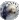Registered User
Join Date
Sep 2010
Location
Martinez, CA
Posts
55
Country Flag: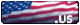## Torque = Hp x 5252 / Rpm (5252 is a constant) - still a valid formula ?

With todays engines where torque output can me manipulated by manufactures through electronics, variable cam timing, etc to be more consistent across wider RPM ranges, is the long held formula of torque = HP x 5252 / RPM still valid?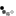Reply With Quote

2.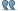Originally Posted by 67-LS1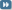With today’s engines where torque output can me “manipulated” by manufactures through electronics, variable cam timing, etc to be more consistent across wider RPM ranges, is the long held formula of torque = HP x 5252 / RPM still valid?
Yes, #becausephysics

AndrewReply With Quote

3.Registered User
Join Date
Sep 2010
Location
Martinez, CA
Posts
55
Country Flag:So how dies one explain torque curves that say reach peak torque at 1800 rpm and hold it constant until 2400 rpm while hp rises from 260 to 300 over the same span?Reply With Quote

4. Variable valve timing, variable vane turbo, advanced engine management strategies.Reply With Quote

5.Registered User
Join Date
Sep 2010
Location
Martinez, CA
Posts
55
Country Flag:So in cases of new engines with new technologies, the original theory is no longer valid?
In my example torque is 258 lb ft at 1800 rpm AND at 2400 rpm.
So....
258 lb ft = 260 HP x 5252 / 1800 RPM. FAIL. Calculation shows 758.6 lb ft
258 lb ft = 300 HP x 5252 / 2400 RPM. FAIL. Calculation shows 656.5 lb ftReply With Quote

6. You are correct. I've never seen a flat torque curve before.Reply With Quote

7.Originally Posted by 67-LS1So how dies one explain torque curves that say reach peak torque at 1800 rpm and hold it constant until 2400 rpm while hp rises from 260 to 300 over the same span?
There is a lot that can be done with today's electronics, variable cam timing, variable turbo boost and direct injection that we could never have dreamed of in the days of carburetors and point type ignition. Torque curves are increasingly flat. That means the horsepower curve is more linear than ever before.

The formula remains true because that is how horsepower is defined. We cannot measure horsepower, only torque and speed. from this, horsepower is calculated.

Most new direct injected and turbocharged cars have very flat torque curves. This is great. It sure beats the big-cammed cars of the 60's and 70's that would not wake up until 3000 rpm and were done at 7000 rpm. Now the problem is getting transmissions to survive under the high torque loads.Reply With Quote

8.Originally Posted by 67-LS1So in cases of new engines with new technologies, the original theory is no longer valid?
In my example torque is 258 lb ft at 1800 rpm AND at 2400 rpm.
So....
258 lb ft = 260 HP x 5252 / 1800 RPM. FAIL. Calculation shows 758.6 lb ft
258 lb ft = 300 HP x 5252 / 2400 RPM. FAIL. Calculation shows 656.5 lb ft
HP = (TQ X RPM) / 5252

An engine can make the same torque at different RPM, what changes is the HP. As RPM climbs, so does HP.

https://spicerparts.com/calculators/...que-calculator
Last edited by andrewb70; 07-08-2019 at 05:48 AM.Reply With Quote

9.Originally Posted by 67-LS1So in cases of new engines with new technologies, the original theory is no longer valid?
In my example torque is 258 lb ft at 1800 rpm AND at 2400 rpm.
So....
258 lb ft = 260 HP x 5252 / 1800 RPM. FAIL. Calculation shows 758.6 lb ft
258 lb ft = 300 HP x 5252 / 2400 RPM. FAIL. Calculation shows 656.5 lb ft
The formula is the ONLY way engine power is calculated. It has not changed, nor will it. The formula is correct, I think your math is off a little.
HP and TQ will ALWAYS be equal at 5252 RPM. This is the way the formula was originally produced, and right or wrong, is how all engines, electric motors, or any rotating device that produce work is measured.

258 lb. ft @ 1800 RPM = 88.4 HP (258 x 1800) / 5252 = HP (464,400) / 5252 = 88.4 HP
258 lb.ft @ 2400 RPM = 117.9 HP (258 x 2400) / 5252 = HP (619,200) / 5252 = 117.9 HP

The formula can be changed to find TQ, but HP and it's RPM must be known.Reply With Quote

10.Registered User
Join Date
Sep 2010
Location
Martinez, CA
Posts
55
Country Flag:Originally Posted by sccacudaThe formula is the ONLY way engine power is calculated. It has not changed, nor will it. The formula is correct, I think your math is off a little.
HP and TQ will ALWAYS be equal at 5252 RPM. This is the way the formula was originally produced, and right or wrong, is how all engines, electric motors, or any rotating device that produce work is measured.

258 lb. ft @ 1800 RPM = 88.4 HP (258 x 1800) / 5252 = HP (464,400) / 5252 = 88.4 HP
258 lb.ft @ 2400 RPM = 117.9 HP (258 x 2400) / 5252 = HP (619,200) / 5252 = 117.9 HP

The formula can be changed to find TQ, but HP and it's RPM must be known.
In my formula, I didn’t make up the given HP or torque numbers. They are all from a torque curve. I can’t figure out how to attach it or I would.
I believe it when you say the formula is tried and true but how does that jibe with engines that have these new flatter curves?
Is this formula for “peak” torque or HP only?
Or is it how HP is “Defined” as Bill states above as opposed to “Measured”?Reply With Quote

11. Torque is what is measured directly. HP is a measure of work performed over time, which is why RPM matters.Reply With Quote

12.Originally Posted by 67-LS1In my formula, I didn’t make up the given HP or torque numbers. They are all from a torque curve. I can’t figure out how to attach it or I would.
I believe it when you say the formula is tried and true but how does that jibe with engines that have these new flatter curves?
Is this formula for “peak” torque or HP only?
Or is it how HP is “Defined” as Bill states above as opposed to “Measured”?
It jibes exactly to the mathematical formulas solution. It is not just tried and true, it is the ONLY way it is measured and is defined by the formula. As I said, your math is off.
I guarantee you that any HP or Tq numbers at a given RPM is derived from this formula, peak or at any other RPM. All dynamometers use this programmed formula to calculate HP and TQ.
Not to be an ass, but this formula is not wrong, it is used by everyone in the world as the only way to correctly measure HP and TQ. When you find where your making your math error, you'll see that it works too...simply because it cannot NOT work!!Reply With Quote

13.Originally Posted by 67-LS1In my formula, I didn’t make up the given HP or torque numbers. They are all from a torque curve. I can’t figure out how to attach it or I would.
I believe it when you say the formula is tried and true but how does that jibe with engines that have these new flatter curves?
Your not understanding something. If your using a TQ curve as a reference, it was calculated with this formula. It doesn't matter if it's flat, peaky, or looks like a mountain range.
Are you sure your not looking at a hybrid vehicle that is factoring in the electric motors TQ into the advertised power output?Reply With Quote

14.Originally Posted by 67-LS1In my formula, I didn’t make up the given HP or torque numbers. They are all from a torque curve. I can’t figure out how to attach it or I would.
I believe it when you say the formula is tried and true but how does that jibe with engines that have these new flatter curves?
Is this formula for “peak” torque or HP only?
Or is it how HP is “Defined” as Bill states above as opposed to “Measured”?
The formula shows the relationship between torque and horsepower at a given engine speed, not at the peak. If you use peak horsepower for the input, you need to also use the rpm at that peak. The same for torque.

Normally peak torque and peak horsepower are not at the same engine speed.Reply With Quote

15.Registered User
Join Date
Sep 2010
Location
Martinez, CA
Posts
55
Country Flag:OK! Figured out how to attach the curve!

I clearly dont understand this, hence my OP and I do appreciate you guys trying to explain it. I know this formula is tried and true and has been since the invention of the horse.

But then I see a curve like the attached and hopefully you can see why I ask the question. The HP curve rises as the torque curve remains flat.Reply With Quote

16. You need to convert your units from kW to HP first.Reply With Quote

17. HP is rising because RPM is rising!Reply With Quote

18. 67 LS1, where did you get your example numbers from? Please double check them for accuracy. Make sure you haven't combined a peak horsepower with a peak torque that occur at different rpm's. Also, make sure that the torque and horsepower scales on the sides of the chart are accurate. Most of the time, torque and horsepower are scaled to use the same Y values on a chart. When torque and HP are scaled differently or use a different set of units (like Newton meters), the chart can be misleading.

67 LS1's math looks correct. As for the formula, it still holds true.

First, let's write the formula in the more familiar form where horsepower is isolated and shown as a result of RPM and torque:

HP = Tq(ft-lbfs)*RPM/5252

Now, let's do some review to appreciate what the units mean.

Force - a push or pull on an object
Energy or work - Force times distance
Power - Energy expended over an amount of time
Torque - rotational force

The relationship should be apparent now. Horsepower is a direct result of the product of torque and rpm, or put more simply, force and speed. More force (or torque) will create more horsepower and more speed (RPM) will create more horsepower just like less of either will reduce horsepower.

Next, let's do a quick unit analysis to demystify the 5252 constant.

1 HP = 550 ft-lbf/sec
1 RPM = 2pi radians/60 seconds

HP = X ft-lbf * Y RPM * (2*pi radians/revolution)*(1min/60 sec) * (1HP/(550 ft-lbf/sec))
HP = X ft-lbf * Y RPM * (2*pi/(33000))*(radians/revolution)*(min/sec)*(HP/(ft-lbf/sec))
HP = X * Y * 5252 HP

This is a physical definition of what is happening. It works for any kind of rotating motor (so long as we're using HP and ft-lbf) whether it's a gas engine, a bike crank, an electric motor, or a kid standing on the end of a lever.Reply With Quote

19.Originally Posted by 67-LS1OK! Figured out how to attach the curve!

I clearly don’t understand this, hence my OP and I do appreciate you guys trying to explain it. I know this formula is tried and true and has been since the invention of the horse.

But then I see a curve like the attached and hopefully you can see why I ask the question. The HP curve rises as the torque curve remains flat.
Because the RPM changed the HP changed. You changed one variable in the formula (RPM) so the result has to change.Reply With Quote

20.Originally Posted by 67-LS1OK! Figured out how to attach the curve!

I clearly don’t understand this, hence my OP and I do appreciate you guys trying to explain it. I know this formula is tried and true and has been since the invention of the horse.

But then I see a curve like the attached and hopefully you can see why I ask the question. The HP curve rises as the torque curve remains flat.
It rises because rpm is increasing. When torque is flat, horsepower increases linearly with rpm. Horsepower is torqueXrpm/5252.

If torque from 1000 to 6000 rpm is 100 ft.lb, then horsepower will be:

1000 rpm - 19 hp
2000 rpm - 38 hp
3000 rpm - 57 hp
4000 rpm - 76 hp
5000 rpm - 95 hp
6000 rpm - 114 hp

Does that make sense now?Reply With Quote